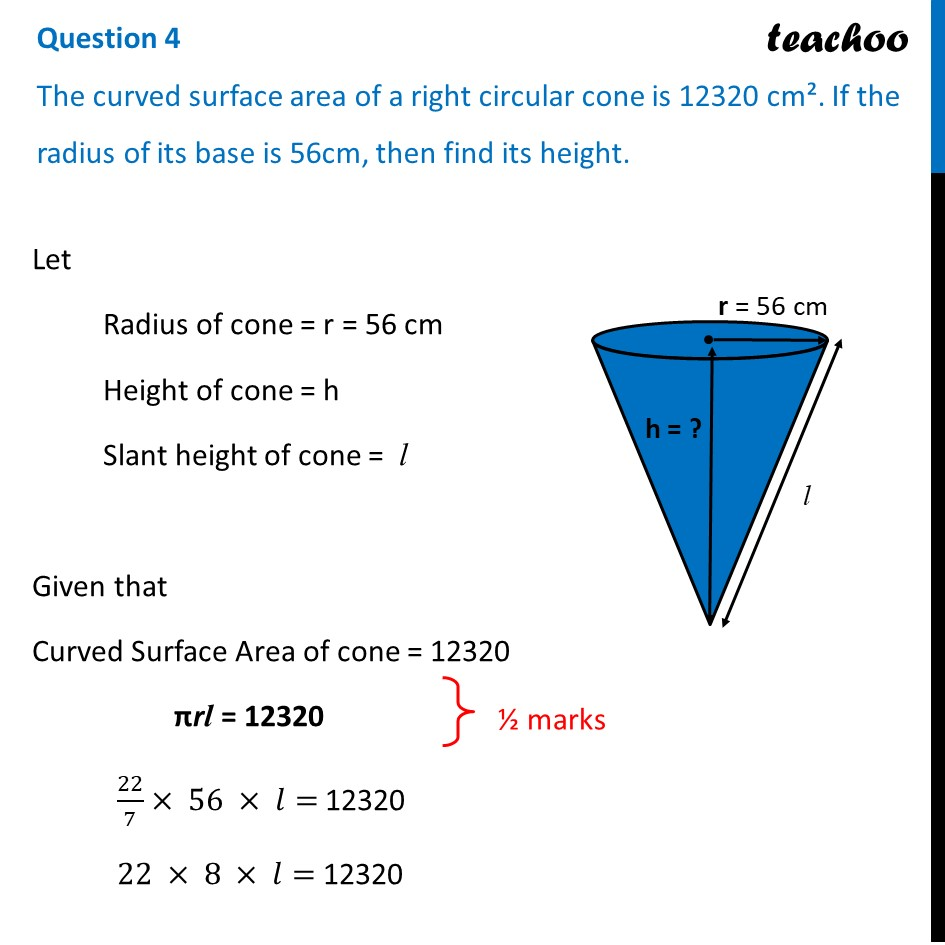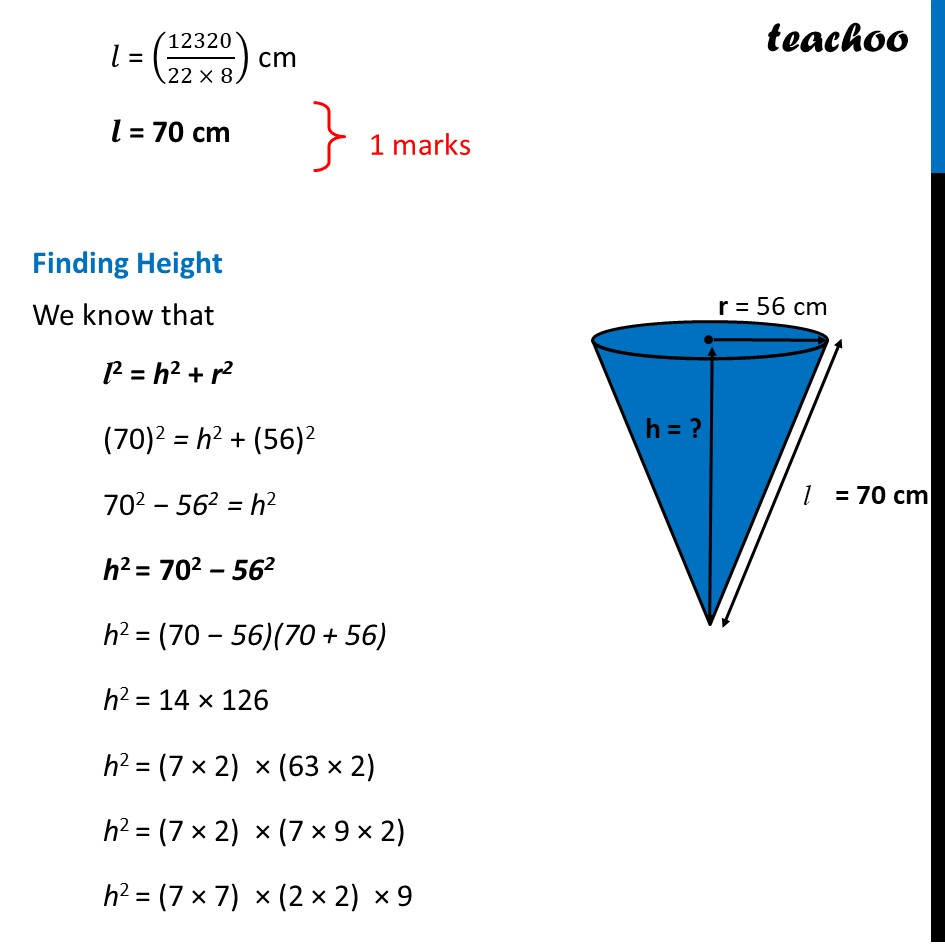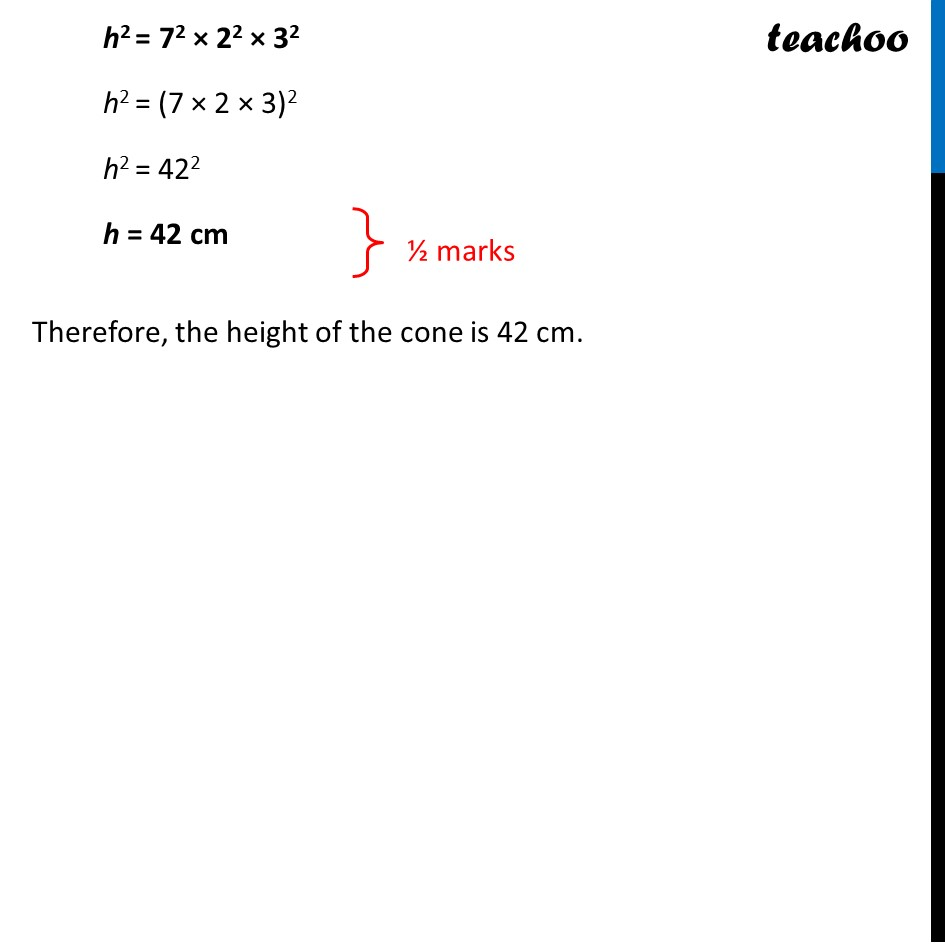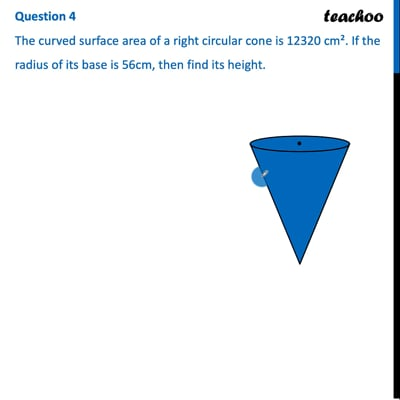CBSE Class 10 Sample Paper for 2022 Boards - Maths Standard [Term 2]

Class 10
Solutions of Sample Papers for Class 10 Boards

## The curved surface area of a right circular cone is 12320 cm². If the radius of its base is 56cm, then find its height.This video is only available for Teachoo black users

### Transcript

Question 4 The curved surface area of a right circular cone is 12320 cm². If the radius of its base is 56cm, then find its height. Let Radius of cone = r = 56 cm Height of cone = h Slant height of cone = l Given that Curved Surface Area of cone = 12320 πrl = 12320 22/7× 56 × 𝑙= 12320 22 × 8 × 𝑙= 12320 𝑙 = (12320/(22 × 8)) cm 𝒍 = 70 cm Finding Height We know that l2 = h2 + r2 (70)2 = h2 + (56)"2" 702 − 562 = h2 h2 = 702 − 562 h2 = (70 − 56)(70 + 56) h2 = 14 × 126 h2 = (7 × 2) × (63 × 2) h2 = (7 × 2) × (7 × 9 × 2) h2 = (7 × 7) × (2 × 2) × 9 h2 = 72 × 22 × 32 h2 = (7 × 2 × 3)2 h2 = 422 h = 42 cm Therefore, the height of the cone is 42 cm.